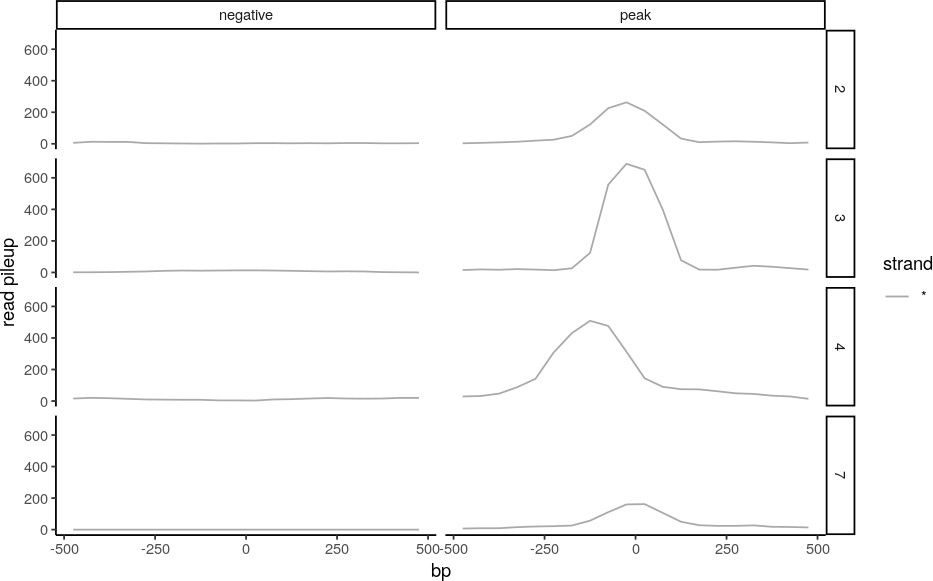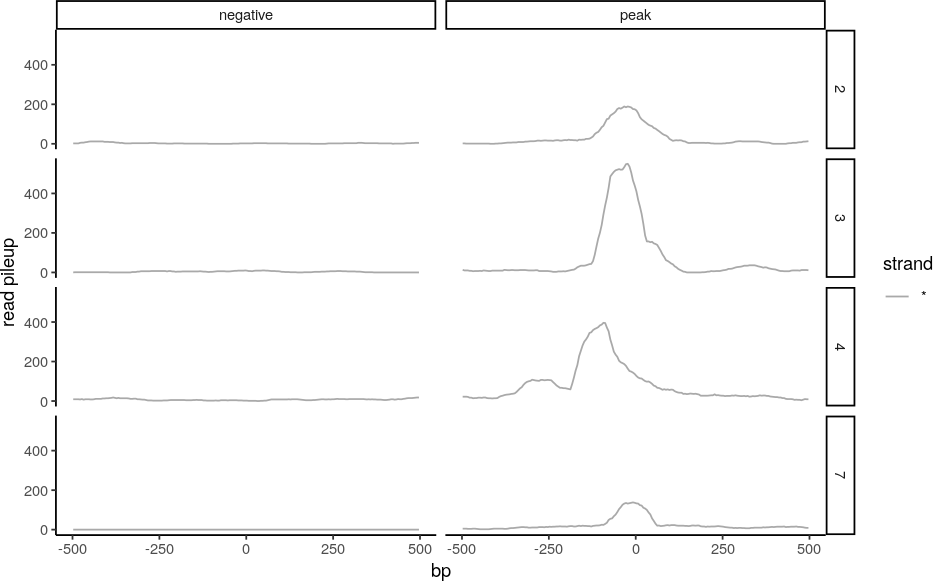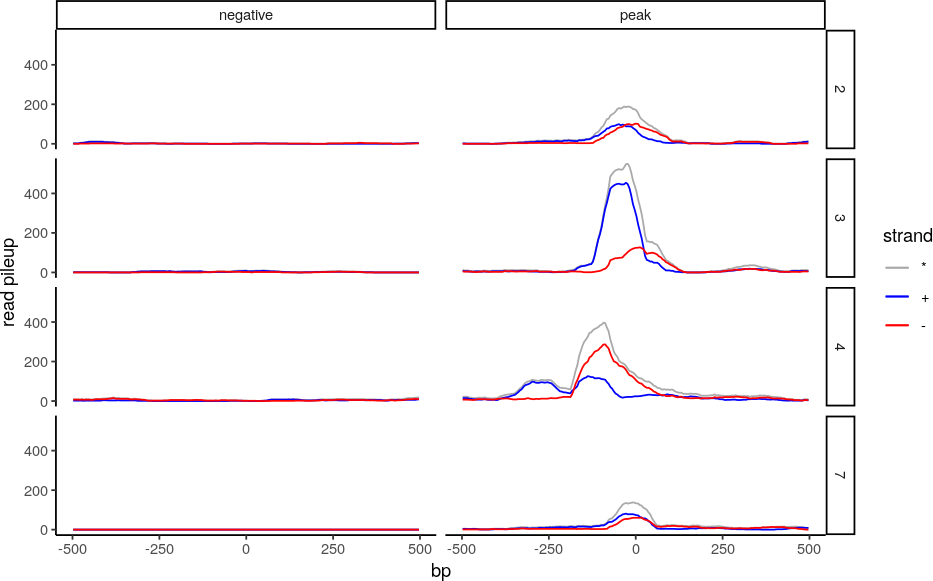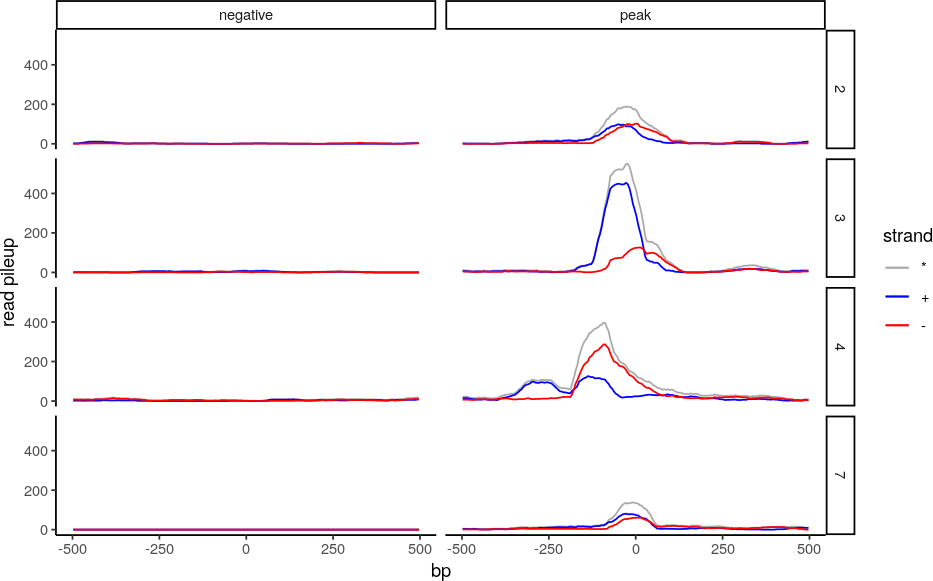# 1 Why bams?

seqsetvis can load profiles from bigWigs or construct profiles of read pileups from bams, which are binary sam files. Binary files are not human readable but are compressed and provide rapid access for computers,

Compared to bam files, bigWigs generally take up much less disk space and are faster to load data from. They are also standard outputs used by many powerful NGS analysis tools (or can be easily generated from bedGraph files) and are accepted as inputs by common genome browsers (UCSC, WashU, IGV etc.).

However, assessing read pileups from bam files directly allows for more flexibilty and is extremely powerful for quality control in ChIP-seq and related data. This vignette will demonstrate methods for loading data from bam files and how the results are useful in assessing ChIP-seq data quality.

``````library(seqsetvis)
library(data.table)
library(GenomicRanges)``````

# 2 The simplest case

A straightforward pileup.

``````bam_dt = ssvFetchBam(bam_file, query_gr, return_data.table = TRUE)
## Warning in load_signal(f, nam, qgr): creating index for /tmp/RtmpKP1IF2/
## Rinst1dd91bd83f6f/seqsetvis/extdata/test_peaks.bam
## fragLen was calculated as: 172
bam_dt[, c("peak_status", "peak_id") := tstrsplit(id, "_", keep = c(4,5))]
p_basic = p_default +
geom_path(data = bam_dt, aes(x = x, y = y, color = strand))
p_basic``````Figure 1: Read pileup with default parameters

``````bam_dt = ssvFetchBam(bam_file,
query_gr,
fragLens = NA,
win_size = 5,
return_data.table = TRUE)
bam_dt[, c("peak_status", "peak_id") := tstrsplit(id, "_", keep = c(4,5))]
p_basic = p_default +
geom_path(data = bam_dt, aes(x = x, y = y, color = strand))
p_basic``````Figure 2: Read pileup with default parameters

``````bam_dt = ssvFetchBam(bam_file,
query_gr,
fragLens = NA,
win_size = 5,
return_data.table = TRUE,
target_strand = "both")
bam_dt[, c("peak_status", "peak_id") := tstrsplit(id, "_", keep = c(4,5))]
p_basic + geom_path(data = bam_dt, aes(x = x, y = y, color = strand)) + facet_grid("peak_id~peak_status")``````# 3 Considering strands and disabling extension to fragment length.

``````bam_dt = ssvFetchBam(bam_file,
win_size = 5,
fragLens = NA,
query_gr,
return_data.table = TRUE,
target_strand = "both")
bam_dt[, c("peak_status", "peak_id") := tstrsplit(id, "_", keep = c(4,5))]
p_basic + geom_path(data = bam_dt, aes(x = x, y = y, color = strand))``````(#fig:basic_NAfragLens)no extension to fragment length

``````bam_dt = ssvFetchBam(bam_file,
win_size = 10,
fragLens = "auto",
query_gr,
return_data.table = TRUE,
max_dupes = 1,
target_strand = "both")